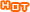# how to say a^2, a^3, a^n in English?

how to say a^2, a^3, a^n in English? For example: a^2+b^3+c^n = d
7 years ago
BPB

a^2: a squared; a^3: a cubed; a^n: a superscript n
Brown

a^2+b^3+c^n = d say: a squared plus b cubed plus c superscript n equals d
Download Từ điển thuật ngữ Tiếng Anh chuyên ngành PDF